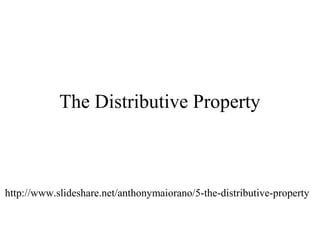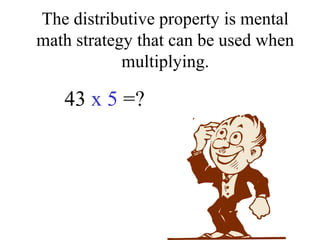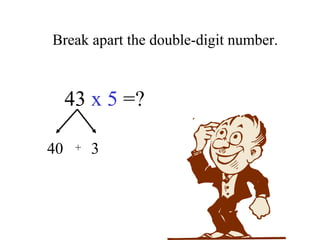Ce diaporama a bien été signalé.
Le téléchargement de votre SlideShare est en cours. ×

# 5 - the distributive propertyChargement dans…3
×

1 sur 108
1 sur 108

# 5 - the distributive property

### 5 - the distributive property

1. 1. The Distributive Property http://www.slideshare.net/anthonymaiorano/5-the-distributive-property
2. 2. The distributive property is mental math strategy that can be used when multiplying. 43 x 5 =?
3. 3. Break apart the double-digit number. 43 x 5 =? 40 3+
4. 4. Then multiply each part by 5. 43 x 5 =? 40 3 x 5 x 5 +
5. 5. Then multiply each part by 5. 43 x 5 =? 40 3 x 5 x 5 200 15 +
6. 6. Finally, sum your two products 43 x 5 =215 40 3 x 5 x 5 200 15+ = 215 +
7. 7. Let’s look at another example. 53 x 6 = ?
8. 8. Break apart the double-digit number. 53 x 6 = ?
9. 9. Break apart the double-digit number. 53 x 6 = ? 50 3+
10. 10. Multiply each part by 6. 53 x 6 = ? 50 3 x 6 x 6 +
11. 11. Multiply each part by 6. 53 x 6 = ? 50 3 x 6 x 6 300 18 +
12. 12. Sum the two products. 53 x 6 = 318 50 3 x 6 x 6 300 + 18 = 318 +
13. 13. There are three steps to the distributive property. 4 x 28 =
14. 14. There are three steps to the distributive property. 4 x 28 = 1) Break apart the double-digit number.
15. 15. There are three steps to the distributive property. 4 x 28 = 4 x (20 + 8) 1) Break apart the double-digit number.
16. 16. There are three steps to the distributive property. 4 x 28 = 4 x (20 + 8) 1) Break apart the double-digit number. 2) Multiply each part by 4. (distribute the 4)
17. 17. There are three steps to the distributive property. 4 x 28 = 4 x (20 + 8) = (4 x 20) + (4 x 8) 1) Break apart the double-digit number. 2) Multiply each part by 4. (distribute the 4)
18. 18. There are three steps to the distributive property. 4 x 28 = 4 x (20 + 8) = (4 x 20) + (4 x 8) 1) Break apart the double-digit number. 2) Multiply each part by 4. (distribute the 4) 3) Sum the two products.
19. 19. There are three steps to the distributive property. 4 x 28 = 4 x (20 + 8) = 80 + (4 x 8) 1) Break apart the double-digit number. 2) Multiply each part by 4. (distribute the 4) 3) Sum the two products.
20. 20. There are three steps to the distributive property. 4 x 28 = 4 x (20 + 8) = 80 + 32 1) Break apart the double-digit number. 2) Multiply each part by 4. (distribute the 4) 3) Sum the two products.
21. 21. There are three steps to the distributive property. 4 x 28 = 4 x (20 + 8) = 80 + 32 = 112 1) Break apart the double-digit number. 2) Multiply each part by 4. (distribute the 4) 3) Sum the two products.
22. 22. The word “distribute” means “to give out.”
23. 23. Distribute the cubes to the girls.
24. 24. Distribute the cubes to the girls.
25. 25. Distribute the cubes to the girls.
26. 26. Distribute the cubes to the girls.
27. 27. Distribute the cubes to the girls.
28. 28. Distribute the cubes to the girls.
29. 29. In this example, the 5 was distributed. 5 x 38 = 5 x (30 + 8) = (5 x 30) + (5 x 8)
30. 30. In this example, the 7 was distributed. 7 x 46 = 7 x (40 + 6) = (7 x 40) + (7 x 6)
31. 31. Find the area of the rectangle. Area = length x width 6 ft 24 ft
32. 32. Find the area of the rectangle. Area = length x width 6 ft 24 ft
33. 33. Find the area of the rectangle. Area = length x width 6 ft 20 ft + 4 ft
34. 34. Find the area of the rectangle. Area = length x width 6 ft 20 ft + 4 ft
35. 35. Find the area of the rectangle. Area = length x width 6 ft 20 ft + 4 ft 6 ft
36. 36. Find the area of the rectangle. Area = length x width 6 ft 20 ft + 4 ft 6 ft Find the area of each rectangle.
37. 37. Find the area of the rectangle. Area = length x width 6 ft 20 ft + 4 ft 6 ft Find the area of each rectangle. 6 x 20 = 120 sq ft
38. 38. Find the area of the rectangle. Area = length x width 6 ft 20 ft + 4 ft 6 ft Find the area of each rectangle. 6 x 20 = 120 sq ft 6 x 4 = 24 sq ft
39. 39. Find the area of the rectangle. Area = length x width 6 ft 20 ft + 4 ft 6 ft Find the area of each rectangle. 120 sq ft 24 sq ft
40. 40. Find the area of the rectangle. Area = length x width 6 ft 20 ft + 4 ft 6 ft Now put the two rectangles back together. 120 sq ft 24 sq ft
41. 41. Find the area of the rectangle. Area = length x width 6 ft 20 ft + 4 ft Now put the two rectangles back together. 120 sq ft 24 sq ft
42. 42. Find the area of the rectangle. Area = length x width 6 ft 20 ft + 4 ft Now put the two rectangles back together. 120 sq ft 24 sq ft
43. 43. Find the area of the rectangle. Area = length x width 6 ft 24 ft Now put the two rectangles back together. 120 sq ft + 24 sq ft
44. 44. Find the area of the rectangle. Area = length x width 6 ft 24 ft Now put the two rectangles back together. 144 sq ft
45. 45. A swimming pool has a shallow end and a deep end. Find the surface area of the pool. shallow water deep water8 yds 5 yds 10 yds
46. 46. shallow water deep water8 yds 5 yds 10 yds 8 yds Break the pool into a deep end and a shallow end.
47. 47. shallow water deep water8 yds 5 yds 10 yds 8 yds Find the area of the deep end.
48. 48. shallow water8 x 5 = 40 8 yds 5 yds 10 yds 8 yds Find the area of the deep end.
49. 49. shallow water8 x 5 = 40 8 yds 5 yds 10 yds 8 yds Find the area of the shallow end.
50. 50. 8 x 10 = 80 8 x 5 = 40 8 yds 5 yds 10 yds 8 yds Find the area of the shallow end.
51. 51. 8 x 10 = 80 8 x 5 = 40 8 yds 5 yds 10 yds 8 yds Now sum the two areas together.
52. 52. 80408 yds 5 yds 10 yds Now sum the two areas together. +
53. 53. 80408 yds 5 yds 10 yds 40 + 80 = 120 square yards
54. 54. Write an expression that shows how to find the area of the rectangle and uses the distributive property. 9 yds 5 yds 20 yds
55. 55. Find the areas for each individual rectangle. 9 yds 5 yds 20 yds
56. 56. Find the areas for each individual rectangle. 9 yds 5 yds 20 yds (9 x 5)
57. 57. Find the areas for each individual rectangle. 9 yds 5 yds 20 yds (9 x 5) (9 x 20)
58. 58. Sum the two areas. 9 yds 5 yds 20 yds (9 x 5) (9 x 20)+
59. 59. (9 x 5) + (9 x 20) = area 9 yds 5 yds 20 yds (9 x 5) (9 x 20)
60. 60. Practice Time
61. 61. 1) Which of the following expressions shows the distributive property for 5 x (3 + 7)? (5 x 3) + (5 x 7) (5 x 3) x (5 x 7) (5 + 3) x (5 + 7)
62. 62. 1) Which of the following expressions shows the distributive property for 5 x (3 + 7)? (5 x 3) + (5 x 7) Correct!
63. 63. 2) Which of the following expressions shows the distributive property for 3 x (9 + 4) ? (3 x 9) + (3 x 4) (3 + 9) + (3 + 4) (3 + 9) x (3 + 4)
64. 64. 2) Which of the following expressions shows the distributive property for 3 x (9 + 4) ? (3 x 9) + (3 x 4) Correct!
65. 65. 3) Which of the following expressions is equivalent to: 2 + 3 + 2 + 3 and shows the distributive property. 2 x (2 + 3) 2 + 2 + 3 + 3 3 x (2 + 3)
66. 66. 3) Which of the following expressions is equivalent to: 2 + 3 + 2 + 3 and uses the distributive property. 2 x (2 + 3) Correct!
67. 67. 4) Which of the following expressions is equivalent to: (4 x 3) + (4 x 8) ? 4 x (3 + 8) 8 x (3 + 4) 3 x (4 + 8)
68. 68. 4) Which of the following expressions is equivalent to: (4 x 3) + (4 x 8) ? 4 x (3 + 8) Correct!
69. 69. 5) Which of the following expressions is equivalent to: (5 x 9) + (5 x 3) ? 9 x (3 + 5) 5 x (9 + 3) 3 x (9 + 5)
70. 70. 5) Which of the following expressions is equivalent to: (5 x 9) + (5 x 3) ? 5 x (9 + 3) Correct!
71. 71. 6) Write an expression that shows how to find the area of the rectangle and uses the distributive property. 4 yds 3 yds 9 yds
72. 72. 6) Write an expression that shows how to find the area of the rectangle and uses the distributive property. 4 yds 3 yds 9 yds
73. 73. 6) Write an expression that shows how to find the area of the rectangle and uses the distributive property. 4 yds 3 yds 9 yds
74. 74. 6) Write an expression that shows how to find the area of the rectangle and uses the distributive property. 4 yds 3 yds 9 yds 4 yds
75. 75. 6) Write an expression that shows how to find the area of the rectangle and uses the distributive property. 4 yds 3 yds 9 yds 4 yds 4 x 3
76. 76. 6) Write an expression that shows how to find the area of the rectangle and uses the distributive property. 4 yds 3 yds 9 yds 4 yds 4 x 3 4 x 9
77. 77. 6) Write an expression that shows how to find the area of the rectangle and uses the distributive property. 4 yds 3 yds 9 yds 4 yds 4 x 3 4 x 9
78. 78. 6) Write an expression that shows how to find the area of the rectangle and uses the distributive property. 4 yds 3 yds 9 yds 4 x 3 4 x 9
79. 79. 6) Write an expression that shows how to find the area of the rectangle and uses the distributive property. 4 yds 3 yds 9 yds 4 x 3 4 x 9
80. 80. 6) Write an expression that shows how to find the area of the rectangle and uses the distributive property. 4 yds 3 yds 9 yds 4 x 3 + 4 x 9
81. 81. 7) Write an expression that shows how to find the area of the rectangle and uses the distributive property. 6 yds 4 yds 8 yds
82. 82. 7) Write an expression that shows how to find the area of the rectangle and uses the distributive property. 6 yds 4 yds 8 yds
83. 83. 7) Write an expression that shows how to find the area of the rectangle and uses the distributive property. 6 yds 4 yds 8 yds
84. 84. 7) Write an expression that shows how to find the area of the rectangle and uses the distributive property. 6 yds 4 yds 8 yds 6 yds
85. 85. 7) Write an expression that shows how to find the area of the rectangle and uses the distributive property. 6 yds 4 yds 8 yds 6 yds 6 x 4
86. 86. 7) Write an expression that shows how to find the area of the rectangle and uses the distributive property. 6 yds 4 yds 8 yds 6 yds 6 x 4 6 x 8
87. 87. 7) Write an expression that shows how to find the area of the rectangle and uses the distributive property. 6 yds 4 yds 8 yds 6 yds 6 x 4 6 x 8
88. 88. 7) Write an expression that shows how to find the area of the rectangle and uses the distributive property. 6 yds 4 yds 8 yds 6 x 4 6 x 8
89. 89. 7) Write an expression that shows how to find the area of the rectangle and uses the distributive property. 6 yds 4 yds 8 yds 6 x 4 6 x 8
90. 90. 7) Write an expression that shows how to find the area of the rectangle and uses the distributive property. 6 yds 4 yds 8 yds 6 x 4 + 6 x 8
91. 91. 8) Write an expression that shows how to find the area of the rectangle and uses the distributive property. 5 yds 2 yds 10 yds
92. 92. 8) Write an expression that shows how to find the area of the rectangle and uses the distributive property. 5 yds 2 yds 10 yds 5 x 2 5 x 10
93. 93. 8) Write an expression that shows how to find the area of the rectangle and uses the distributive property. 5 yds 2 yds 10 yds 5 x 2 + 5 x 10
94. 94. 9) Write an expression that shows how to find the area of the rectangle and uses the distributive property. 8 yds 3 yds 5 yds
95. 95. 9) Write an expression that shows how to find the area of the rectangle and uses the distributive property. 8 yds 3 yds 5 yds 8 x 3 8 x 5
96. 96. 9) Write an expression that shows how to find the area of the rectangle and uses the distributive property. 8 yds 3 yds 5 yds 8 x 3 + 8 x 5
97. 97. 10) Write an expression that shows how to find the area of the rectangle and uses the distributive property. 5 yds x yds 10 yds
98. 98. 10) Write an expression that shows how to find the area of the rectangle and uses the distributive property. 5 yds x yds 10 yds 5x 5 ∙ 10
99. 99. 10) Write an expression that shows how to find the area of the rectangle and uses the distributive property. 5 yds x yds 10 yds 5x + 5 ∙ 10
100. 100. 11) Which expression is equivalent to 3(x + 7)? 3x + 7 x + 21 x + 10 3x + 21
101. 101. 11) Which expression is equivalent to 3(x + 7)? 3x + 21 Correct!
102. 102. 12) Which expression is equivalent to 4(x + 5)? 4x + 5 4x + 20 x + 9 9x
103. 103. 12) Which expression is equivalent to 4(x + 5)? 4x + 20 Correct!
104. 104. 13) Which expression is equivalent to 8(x + 2)? 8x + 16 8x + 2 10x 8x + 10
105. 105. 13) Which expression is equivalent to 8(x + 2)? 8x + 16 Correct!
106. 106. 14) Which expression is equivalent to 2(x + 3)? 2x + 3 2x + 5 2x + 6 2x + 2
107. 107. 14) Which expression is equivalent to 2(x + 3)? 2x + 6 Correct!
108. 108. The End.